# matplotlib画图教程系列之-堆积柱状图

## matplotlib堆积柱状图画法介绍

# -*- coding:utf-8 -*-
import pandas as pd
import matplotlib.pyplot as plt
import numpy as np
df.fillna(value={'mass':df.mass.mean()},inplace=True)
temp = pd.pivot_table(data=df,index='method',columns='number',values='mass',aggfunc=np.sum)
print(temp)
temp.fillna(0,inplace=True)
print(temp.columns.values)#获取dataframe 的x方向的属性值
plt.rcParams['font.sans-serif']=['SimHei']#这两句作用为防止中文乱码
plt.rcParams['axes.unicode_minus']=False
tick_label=['数量1','数量2','数量3','数量4','数量5','数量6','数量7'])
plt.bar(x=temp.columns.values,height=temp.loc['Transit',:],color='red',label='Transit', \
plt.legend(loc='best')
plt.show()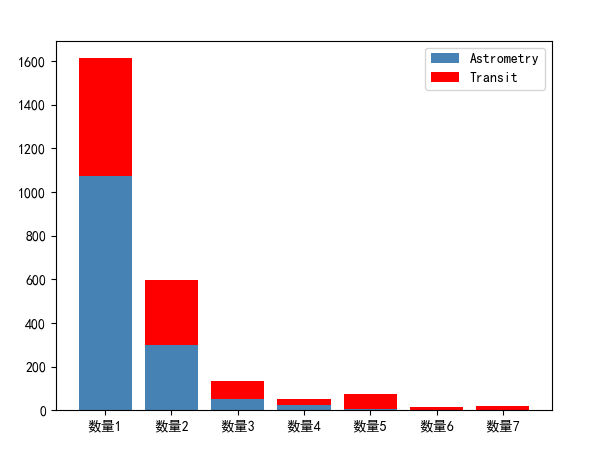## 补充细节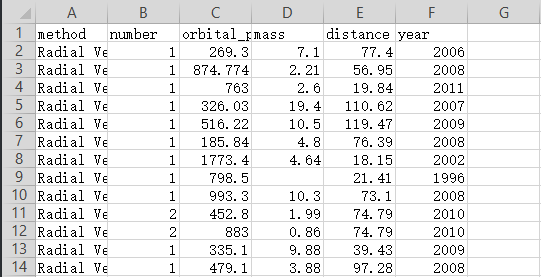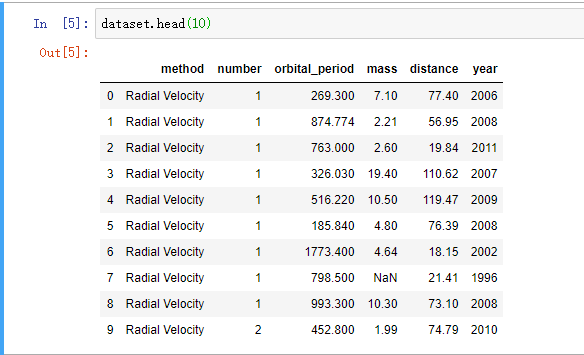pandas.pivot_table() 这个方法叫做透视表。

pandas.pivot_table(index,columns,values)

• index 选择属性作为行
• columns 选择属性作为列
• values 选择所要查看的结果值

0 one A 1 x

1 one A 2 y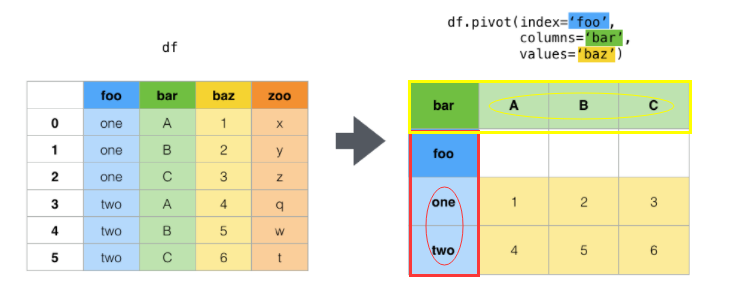index=‘foo’ 表示将’foo’这一列所有可取值作为行名

columns=‘bar’ 表示将’bar’这一列所有可取值作为右边的列名

values=‘baz’ 表示将’baz’这一列的值作为最终显示结果。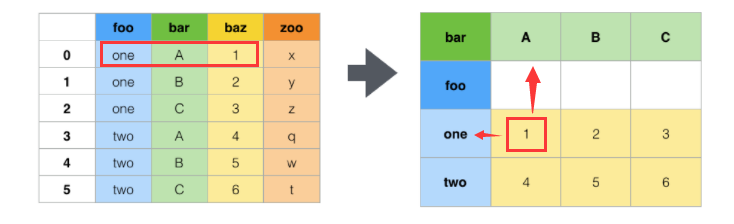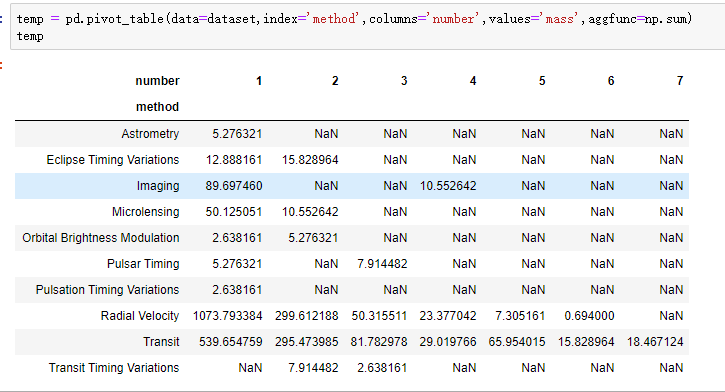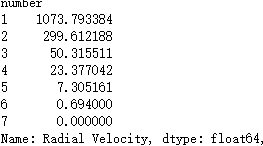temp.loc[‘Transit’,:]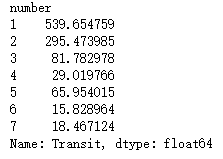10-166410
06-241万+
10-011万+
06-201万+
01-29263
09-051207
11-2273
09-18
12-09137
10-102920
01-211520
06-122635
04-06484
03-017091
09-01410
11-134249